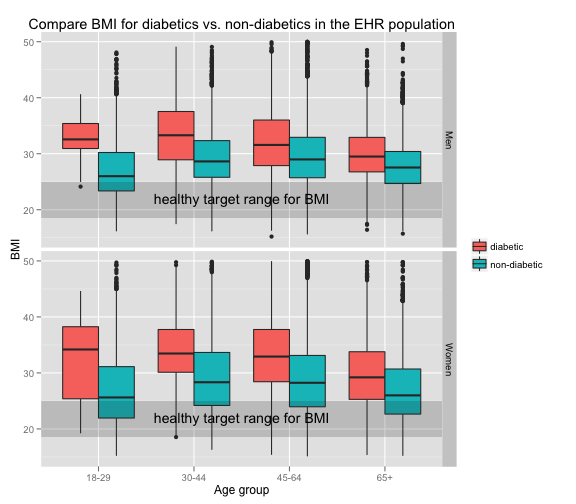# Test run

``````rm(list = ls())
library(ggplot2)
library(scales)
library(car)
``````
``````## Loading required package: MASS
``````
``````## Loading required package: nnet
``````
``````
# pull data in
# do some data clean up
d2\$Height = as.numeric(d2\$Height)
d2\$dmIndicator = as.logical(d2\$dmIndicator)

d2\$age = d2\$VisitYear - d2\$YearOfBirth
d2\$age[d2\$age < 10] = NA
d2\$age.bin = cut(as.numeric(d2\$age), c(10, 30, 45, 65, 100), c("18-29", "30-44",
"45-64", "65+"))
d2\$Gender2 = recode(d2\$Gender, "'M'='Men'; 'F'='Women'; else=NA")
d2\$dmIndicator2 = recode(d2\$dmIndicator, "TRUE='diabetic'; FALSE='non-diabetic'; else=NA")
``````
``````## Warning: NAs introduced by coercion
``````
``````d2.ss = subset(d2, Weight < 400 & Weight > 60 & Height > 48 & Height < 96)
``````

And a plot

``````p <- qplot(age.bin, BMI, data = subset(d2.ss, !is.na(age.bin)), fill = dmIndicator2,
geom = "boxplot", position = "dodge", ylim = c(15, 50), aes(linetype = "dotted"),
main = "Compare BMI for diabetics vs. non-diabetics in the EHR population") +
facet_grid(Gender2 ~ .)
p + annotate("rect", ymin = 18.5, ymax = 25, xmin = -Inf, xmax = Inf, alpha = 0.2) +
labs(x = "Age group", y = "BMI", fill = "") + annotate("text", x = 2.5,
y = 22, label = "healthy target range for BMI")
``````
``````## Warning: NAs introduced by coercion
``````
``````## Warning: NAs introduced by coercion
``````
``````## Warning: Removed 70 rows containing non-finite values (stat_boxplot).
``````
``````## Warning: Removed 381 rows containing non-finite values (stat_boxplot).
``````